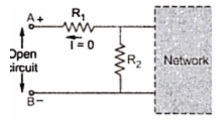### Short and Open Circuits

In the network simplification, short circuit or open circuit existing in the network plays an important role.
1. Short Circuit
When any tow points in a network are joined directly to each other with a thick metalic conducting wire, the tow points are said to be short circuited. The resistance of such short circuit is zero.
The part of the network, which is short circuited is shown in the Fig. 1. The points A and B are short circuited. The resistance of the branch A-B is  Rsc = 0 Ω.Fig. 1   Short circuit

The current IAB  is flowing through the short circuited path.
According to Ohm's law,
VAB  = Rsc x IAB  = 0 x IAB  = 0 V
Key point : Thus, voltage across short circuit is always zero through current flows though the short circuited path.

2. Open Circuit
Where there is no connection between the tow points of a network, having some voltage across the tow points then the tow points are said to be open circuited.
As there is no direct connection in an open circuit, the resistance of the open circuit is
The part of the network which is open circuited is shown in the Fig. 2. The points A and B are said to be open circuited. The resistance of the branch AB is ROC = ∞ Ω.Fig. 2  Open circuit

There exists a voltage across the points AB called  open circuit voltage,VAB but ROC = ∞ Ω.
According to Ohm's law,

Key point : Thus, current through open circuit is always zero though there exist a voltage across open circuited terminals.

3. Redundant Branches and Combinations
The redundant means excessive and unwanted.
Key point : If an a circuit there are branches or combinations of elements which do not carry any current then such branches and combinations are called redundant from circuit point of view.
The redundant branches and combinations can be removed and these branches do not affect the performance of the circuit.
The tow important situations of redundancy which may exist in practical circuit are,
Situation 1 : Any branch or combination across which there exist a short circuit, becomes redundant as it does not carry any current.
If in a network, there exists a direct short circuit across a resistance or the combination of resistances then that resistance or the entire combination of resistances becomes inactive from the circuit point of view.
Such A combination is redundant from circuit point of view.
To understand this, consider the combination of resistances and a short circuit as shown in the Fig. 3(a) and (b).Fig . 3  Redundant branches

In Fig. 3(a), there is short circuit across R3. The current always prefers low resistance path hence entire current I passes through short circuit and hence resistance R3 becomes redundant from the circuit point of view.
In Fig. 3(b), there is short circuit across combination of R3 and R4. The entire current flows through short circuit across R3 and R4 and no current can flow through combination of R3 and R4. Thus that combination becomes meaningless from the circuit point of view. Such combinations can be eliminated while analysing the circuit.
Situation 2 : If these is open circuit in a branch or combination, it can not carry any current and becomes redundant.
In Fig. 4 as there exists open circuit branch BC, the branch BC and CD can not carry any current and are become redundant from circuit point of view.Fig.  4  Redundant branches due to open circuit